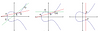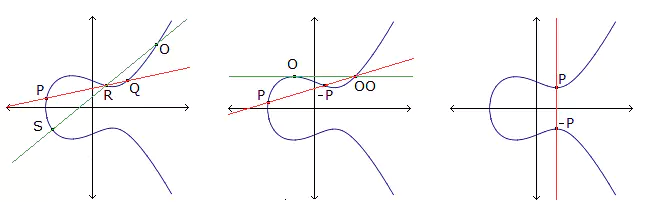# Linear nonbinary covering codes and saturating sets in projective spaces

### Journal article

A. Davydov, M. Giulietti, S. Marcugini, F. Pambianco

Semantic Scholar ArXiv DBLP DOI
Cite

### Cite

APA
Davydov, A., Giulietti, M., Marcugini, S., & Pambianco, F. (2009). Linear nonbinary covering codes and saturating sets in projective spaces. Adv. Math. Commun.

Chicago/Turabian
Davydov, A., M. Giulietti, S. Marcugini, and F. Pambianco. “Linear Nonbinary Covering Codes and Saturating Sets in Projective Spaces.” Adv. Math. Commun. (2009).

MLA
Davydov, A., et al. “Linear Nonbinary Covering Codes and Saturating Sets in Projective Spaces.” Adv. Math. Commun., 2009.

## Abstract

Let $\mathcal A$R,q denote a family of covering codes, in which the covering radius $R$ and the size $q$ of the underlying Galois field are fixed, while the code length tends to infinity. The construction of families with small asymptotic covering densities is a classical problem in the area of Covering Codes.    In this paper, infinite sets of families $\mathcal A$R,q, where $R$ is fixed but $q$ ranges over an infinite set of prime powers are considered, and the dependence on $q$ of the asymptotic covering densities of $\mathcal A$R,q is investigated. It turns out that for the upper limit $\mu$q*(R,$\mathcal A$R,q) of the covering density of $\mathcal A$R,q, the best possibility is $\mu$q*(R,$\mathcal A$R,q)=$O(q)$. The main achievement of the present paper is the construction of optimal infinite sets of families $\mathcal A$R,q, that is, sets of families such that relation $\mu$q*(R,$\mathcal A$R,q)=$O(q)$ holds, for any covering radius $R\geq 2$.    We first showed that for a given $R$, to obtain optimal infinite sets of families it is enough to construct $R$ infinite families $\mathcal A$R,q(0), $\mathcal A$R,q(1), $\ldots$, $\mathcal A$R,q(R-1) such that, for all $u\geq u$0, the family $\mathcal A$R,q($\gamma$) contains codes of codimension $r$u$=Ru + \gamma$ and length $f$q($\gamma$)($r$u) where $f$q($\gamma$)$(r)=O(q$(r-R)/R$)$ and $u$0 is a constant. Then, we were able to construct the necessary families $\mathcal A$R,q($\gamma$) for any covering radius $R\geq 2$, with $q$ ranging over the (infinite) set of $R$-th powers. A result of independent interest is that in each of these families $\mathcal A$R,q($\gamma$), the lower limit of the covering density is bounded from above by a constant independent of $q$.    The key tool in our investigation is the design of new small saturating sets in projective spaces over finite fields, which are used as the starting point for the $q$m-concatenating constructions of covering codes. A new concept of $N$-fold strong blocking set is introduced. As a result of our investigation, many new asymptotic and finite upper bounds on the length function of covering codes and on the smallest sizes of saturating sets, are also obtained. Updated tables for these upper bounds are provided. An analysis and a survey of the known results are presented.

Share